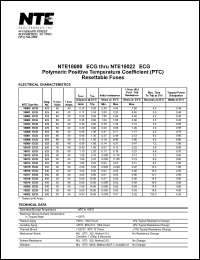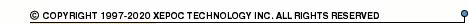More than4 307 195 components listedNTE16006 series datasheets. Manufacturer: NTE.

 NTE16000-ECG Polymetric positive temperature coefficient (PTC) resettable fuse. in 2-pin Diagram 629 package. Operational temperature range from -40°C to 85°C. Datasheet*) NTE16001-ECG Polymetric positive temperature coefficient (PTC) resettable fuse. in 2-pin Diagram 629 package. Operational temperature range from -40°C to 85°C. Datasheet*) NTE16002-ECG Polymetric positive temperature coefficient (PTC) resettable fuse. in 2-pin Diagram 629 package. Operational temperature range from -40°C to 85°C. Datasheet*) NTE16003-ECG Polymetric positive temperature coefficient (PTC) resettable fuse. in 2-pin Diagram 629 package. Operational temperature range from -40°C to 85°C. Datasheet*) NTE16004-ECG Polymetric positive temperature coefficient (PTC) resettable fuse. in 2-pin Diagram 629 package. Operational temperature range from -40°C to 85°C. Datasheet*) NTE16005-ECG Polymetric positive temperature coefficient (PTC) resettable fuse. in 2-pin Diagram 629 package. Operational temperature range from -40°C to 85°C. Datasheet*) NTE16006-ECG Polymetric positive temperature coefficient (PTC) resettable fuse. in 2-pin Diagram 629 package. Operational temperature range from -40°C to 85°C. Datasheet*) NTE16007-ECG Polymetric positive temperature coefficient (PTC) resettable fuse. in 2-pin Diagram 629 package. Operational temperature range from -40°C to 85°C. Datasheet*) NTE16008-ECG Polymetric positive temperature coefficient (PTC) resettable fuse. in 2-pin Diagram 629 package. Operational temperature range from -40°C to 85°C. Datasheet*) NTE16009-ECG Polymetric positive temperature coefficient (PTC) resettable fuse. in 2-pin Diagram 629 package. Operational temperature range from -40°C to 85°C. Datasheet*) NTE16010-ECG Polymetric positive temperature coefficient (PTC) resettable fuse. in 2-pin Diagram 630 package. Operational temperature range from -40°C to 85°C. Datasheet*) NTE16011-ECG Polymetric positive temperature coefficient (PTC) resettable fuse. in 2-pin Diagram 629 package. Operational temperature range from -40°C to 85°C. Datasheet*) NTE16012-ECG Polymetric positive temperature coefficient (PTC) resettable fuse. in 2-pin Diagram 629 package. Operational temperature range from -40°C to 85°C. Datasheet*) NTE16013-ECG Polymetric positive temperature coefficient (PTC) resettable fuse. in 2-pin Diagram 629 package. Operational temperature range from -40°C to 85°C. Datasheet*) NTE16014-ECG Polymetric positive temperature coefficient (PTC) resettable fuse. in 2-pin Diagram 629 package. Operational temperature range from -40°C to 85°C. Datasheet*) NTE16015-ECG Polymetric positive temperature coefficient (PTC) resettable fuse. in 2-pin Diagram 630 package. Operational temperature range from -40°C to 85°C. Datasheet*) NTE16016-ECG Polymetric positive temperature coefficient (PTC) resettable fuse. in 2-pin Diagram 630 package. Operational temperature range from -40°C to 85°C. Datasheet*) NTE16017-ECG Polymetric positive temperature coefficient (PTC) resettable fuse. in 2-pin Diagram 630 package. Operational temperature range from -40°C to 85°C. Datasheet*) NTE16018-ECG Polymetric positive temperature coefficient (PTC) resettable fuse. in 2-pin Diagram 630 package. Operational temperature range from -40°C to 85°C. Datasheet*) NTE16019-ECG Polymetric positive temperature coefficient (PTC) resettable fuse. in 2-pin Diagram 630 package. Operational temperature range from -40°C to 85°C. Datasheet*) NTE16020-ECG Polymetric positive temperature coefficient (PTC) resettable fuse. in 2-pin Diagram 630 package. Operational temperature range from -40°C to 85°C. Datasheet*) NTE16021-ECG Polymetric positive temperature coefficient (PTC) resettable fuse. in 2-pin Diagram 630 package. Operational temperature range from -40°C to 85°C. Datasheet*) NTE16022-ECG Polymetric positive temperature coefficient (PTC) resettable fuse. in 2-pin Diagram 630 package. Operational temperature range from -40°C to 85°C. Datasheet*) NTE16006 Silicon NPN transistor. Low frequency output amp. High current gain. in 3-pin M type mold package. Operational temperature range from 0°C to 150°C. Datasheet*)